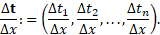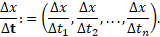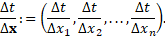iSoul In the beginning is reality.

# Tag Archives: Space & Time

Matters relating to length and duration in physics and transportation

# 4D Formulations of Newtonian Mechanics

Four-Dimensional Formulations of Newtonian Mechanics

First we reproduce section 2 from Michael Friedman’s “Simultaneity in Newtonian Mechanics and Special Relativity” in Foundations of Space-Time Theories (ed. Earman et al., UMinn, 1977), p.405-407. Then we provide the dual.

According to the spatio-temporal point of view, the basic object of both our theories is a four-dimensional manifold. I shall use R4, the set of quadruples of real numbers, to represent the spatio-temporal manifold. Both theories agree that there is a natural system of straight lines defined on this manifold. If (a0, a1, a2, a3), (b0, b1, b2, b3) are two fixed points in R4, then a straight line is a subset of R4 consisting of elements (x0, x1, x2, x3) of the form

(1) x0 = a0r + b0
x1 = a1r + b1
x2 = a2r + b2
x3 = a3r + b3

where r ranges through the real numbers. A curve on R4 is a (suitably continuous and differentiable) map σ: R → R4. Such a curve σ(u) is a geodesic if and only if it satisfies

(2) x0 = a0u + b0
x1 = a1u + b1
x2 = a2u + b2
x3 = a3u + b3

where (x0, x1, x2, x3) = σ(u) and the ai and bi are constants. So if a curve is a geodesic its range is a straight line. Note that the geodesies are just the curves that satisfy

(3) d2xi/du2 = 0       i = 0, 1, 2, 3.

The importance of straight lines and geodesies is due to the fact that both theories agree that the trajectories of free particles are spatio-temporal straight lines. So we can represent such trajectories as geodesies in R4.

# Note

This blogger is focused on developing a new six-dimensional theory of time (an early version is posted here). The glossary is also being updated (see here). Good progress is being made, with the double frame and three-dimensional time aspect especially new. RG

# Temporo-spatial Newtonian mechanics

We follow the treatment by David Tong of Cambridge University in his Classical Dynamics.

A tempicle is defined as a moving object of insignificant time. The motion of a tempicle of vass n at the chronation t is governed by Newton’s Temporo-spatial Second Law, R = nb or, more precisely,

R(t; t′) = h′           (1.1)

where R is the release which, in general, can depend on both the chronation t as well as the lenticity t′, and h = nt′ is the fulmentum. Both R and h are 3-vectors which we denote by the bold font. A prime indicates differentiation with respect to stance x. Equation (1.1) reduces to R = nb if n′ = 0. But if n = n(x), then the form with h′ is correct.

General theorems governing differential equations guarantee that if we are given t and t′ at an initial stance x = x0, we can integrate equation (1.1) to determine t(x) for all x (as long as R remains finite). This is the goal of classical dynamics.

# Composition order

Written compositions organized by temporal order are narratives. Items such as descriptions of people, places, or objects are organized as they occur to the narrator, for example, as the narrator takes apart an object or walks through a building or meets various people. This is a common method of composition but there are others.

Spatial order is another method of composition. Items such as descriptions of people, places, or objects are organized by their physical or spatial positions or relationships, for example, starting at the top and proceeding downward. Explanations of a geopolitical matter might proceed in geographic order.

Travel can be described temporally or spatially. An itinerary is usually arranged temporally but telling about it afterwards might be more interesting if arranged spatially. There are other principles of organization such as climactic order (order of importance) and topical order.

In science the independent variable determines the type of organization. If the independent variable is time, the organization is temporal. If the independent variable is space or distance, the organization is spatial. The stance in spatial organization corresponds to the time in temporal organization.

The values of the independent variable are the index to the order of the composition. If the independent variable is time, then the times indicate the steps in the order. If the independent variable is space or distance, then the stances indicate the steps in the order. Once the step is indicated, the composition may be the same: whether it’s Tuesday, so the tour is in Paris or it’s Paris, so the tour is on Tuesday makes no difference.

# Equations of Motion Generalized

This is an update and expansion of the post here.

Here is a derivation of the spatio-temporal equations of motion, in which acceleration is constant. Let time = t, location = x, initial location = x(t0) = x0, velocity = v, initial velocity = v(t0) = v0, speed = v = |v|, and acceleration = a.

First equation of motion

v = ∫ a dt = v0 + at

Second equation of motion

x = ∫ (v0 + at) dt = x0 + v0t + ½at²

Third equation of motion

From v² = vv = (v0 + at) ∙ (v0 + at) = v0² + 2t(av0) + a²t², and

(2a) ∙ (xx0) = (2a) ∙ (v0t + ½at²) = 2t(av0) + a²t² = v² ‒ v0², it follows that

v² = v0² + 2(a ∙ (xx0)), or

v² − v0² = 2ax, with x0 = 0.

Here is a derivation of the temporo-spatial equations of motion, in which retardation is constant. Let stance = x, time (chronation) = t, initial time = t(x0) = t0, lenticity = w, initial lenticity = w(x0) = w0, pace w = |w|, and retardation = b.

# A theory of 6D space and time

Note: as the research develops this post will be updated.

Introduction

Experience shows motion takes place in three dimensions. There are two measures of the extent of motion: length and duration. The length of motion in three dimensions comprises three-dimensional space. The duration of motion in three dimensions comprises three-dimensional time. Length and duration are symmetric concepts, as will be shown below.

Introduction

An independent variable is specified prior to measuring any dependent variable, so an independent variable is the domain of a functionally-related dependent variable. The independent variable is commonly an interval of time. Distance is the independent variable of an inverse square law. In Hooke’s law the independent variable is mass.

A date-time or time-stamp is a combined time-of-day and date on the calendar, which is of interest in history and astronomy. A time interval or elapsed time is the difference between two date-times, which is of interest in science.

A linear reference is of interest in geography and transportation. The stance interval or distance is the difference between two linear references, which is of interest in science.

Distance is an equivalence relation between pairs of (spatial) points. Distime is an equivalence relation between pairs of instants.

An elapsed time or distime is the date-time that changes during an event or motion. A travel stance or distance is the change in linear reference during an event or motion.

Variables of time periods and distances are fixed. Variables of elapsed values are increasing from a starting point. Intervals are deltas of elapsed values. E.g., time periods are deltas of time. Distances are deltas of stances, that is, stations or points along a line or curve. Elapsed time and elapsed distance, or stance, are increasing variables.

Given that there are three dimensions of motion, and that every motion is measured by its length and duration, then motion requires three dimensions of length and three dimensions of duration. Three dimensions of length are called three-dimensional space. Three dimensions of duration are called three-dimensional time.

For example, motion on a two-dimensional surface can be presented as a two-dimensional map scaled in units of length or as a two-dimensional map scaled in units of duration. [The latter time maps are …]

# What is a clock?

What is a clock? it is a device that measures time, but what are the essentials of a clock? I submit these are the essentials of a clock:

(1) A clock requires a uniform motion. Because only the kinematics (not the dynamics) are significant, a uniform rotation is acceptable. But because the result will be represented as a line – a timeline or time axis – a linear uniform motion has a more direct connection with what is measured, so let us take the first essential as a uniform linear motion.

(2) In order for clocks to be measuring alike, it is necessary that there be a standard rate for all clocks. In addition, clocks should have a standardized beginning point, so that clocks are interchangeable.

(3) A clock requires a pointer which indicates the present time on a time scale as it moves at the standard uniform rate. This would be the hands and dial on a common analogue clock. On a linear clock it is a part whose position in motion is interpreted as the present value of time. The pointer and scale are essentials of a clock.

Furthermore, a clock must be interpreted as showing the present time of the observer’s rest frame.

All the essentials of a clock can be represented by a frame in standard uniform motion relative to the observer’s rest frame. In that case, a clock should be definable in terms of frames of reference: one rest frame and one frame in uniform motion relative to the observer’s rest frame, as in the following.

# Space, time, and dimension

The post continues the ones here, here, and here.

There are three dimensions of motion. The extent of motion in each dimension may be measured by either length or time (duration). There are three dimensions of length and three dimensions of time (duration) for a total of six dimensions.

But there is no six-dimensional metric. Why? Because a metric requires all dimensions to have the same units, which requires a ratio to convert one unit into the other unit. The denominator on a ratio is a one-dimensional quantity, which means either the length or time dimensions need to be reduced by two dimensions.

This ratio is a conversion factor that is either a speed, which multiplied by a time equals a length, or a pace, which multiplied by a length equals a time. In general a speed is the ratio Δdr²/|Δdt²| = Δdr²/(Δt1² + Δt2² + Δt3²)1/2, and a pace is the ratio Δdt²/|Δdr²| = Δdt²/(Δx² + Δy² + Δz²)1/2. The denominator is a distance or distime, which is a linear measure of length or time (duration).

The conversion factors required are the speed of light in a vacuum, c, or its inverse, the pace of light in a vacuum, k. The resulting four-dimensional metric is either c²dt² − dx² − dy² − dz² (with time reduced to one dimension) or dt1² − dt2² − dt3² − k²dr² (with space reduced to one dimension).

These metrics are often simplified by taking c = 1 and k = 1 so that symbolically they are the same. Their units are not the same, however.

Each metric may be further reduced by separating space and time, as in classical physics. Then the space metric is |Δdr²| = (Δx² + Δy² + Δz²)1/2 and the time metric is |Δdt²| = (Δt1² + Δt2² + Δt3²)1/2. In the classical (3+1) of three space dimensions and one time dimension, time is replaced by its metric, and in the classical (1+3) of one space dimension and three time dimensions, space is replaced by its metric.

# Space and time as frames

An observer is a body capable of use as a measurement apparatus. An inertial observer is an observer in inertial motion, i.e., one that is not accelerated with respect to an inertial system. An observer here shall mean an inertial observer.

An observer makes measurements relative to a frame of reference. A frame of reference is a physical system relative to which motion and rest may be measured. An inertial frame is a frame in which Newton’s first law holds (a body either remains at rest or moves in uniform motion, unless acted upon by a force). A frame of reference here shall mean an inertial frame.

A rest frame of observer P is a frame at rest relative to P. A motion frame of observer P is a frame in uniform motion relative to P. Each observer has at least one rest frame and at least one motion frame associated with it. An observer’s rest frame is three-dimensional, but their motion frame is effectively one-dimensional, that is, only one dimension is needed.

Space is the geometry of places and lengths in R3. A place point (or placepoint) is a (spatial) point. The space origin is a reference place point. The location of a place point is the space vector to it from the space origin. Chron (3D time) is the geometry of times and durations in R3. A time point (or timepoint) is an instant. The time origin is a reference time point. The chronation of a time point is the chron vector to it from the time origin.

A frame of reference is unmarked if there are no units specified for its coordinates. A frame of reference is marked by specifying (1) units of either length or duration for its coordinates and (2) an origin point. A space frame of observer P is a rest frame of P that is marked with units of length. A time frame of observer P is a motion frame of P that is marked with units of duration.

Speed, velocity, and acceleration require an independent motion frame. Pace, lenticity, and retardation require an independent rest frame. These independent frames are standardized as clocks or odologes so they are the same for all observers.

Let there be a frame K1 with axes a1, a2, and a3, that is a rest frame of observer P1, and let there be a motion frame K2 with axes 1, 2, and 3, that is a motion frame of P1 along the coincident a1-a´1 axis. See Figure 1.

# Vectors and functions for space and time

The time velocity of an n-dimensional vector variable or vector-valued function Δx per unit of an independent scalar variable t equalsSimilarly, the space lenticity with Δt and Δx, respectively:The rate of a scalar variable Δx per unit of an independent n-dimensional vector variable or vector-valued function Δt equalsThe same except with Δt and Δx, respectively: